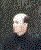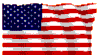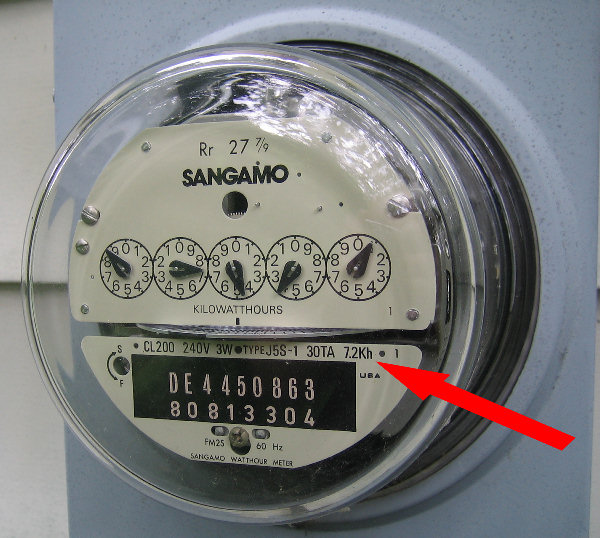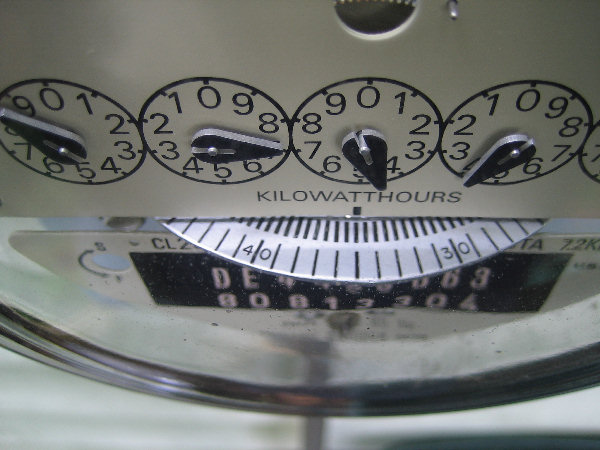www.ChuckLohr.comThis is a non-commercial web site.  Advertising is not accepted.  I am not being paid for any mention or promotion.

HOW TO MEASURE THE WATTAGE OF HOME APPLIANCES

 You can use your home service entrance electric meter to measure the wattage of your home, or the wattage of one device or appliance in your home.

by Charles Lohr         last edited August 25, 2018Meter Motion

 Summary: Use the meter constant (i.e. 7.2) printed on the face of the meter (c). Measure the time in seconds for the metal disk to make one revolution (t). Wattage = (c * 3600) / t.

1. METER CONSTANT  - Get the meter constant (c) from the face of the meter (see the RED ARROW). In this example c is 7.2Kh. This means 7.2 watt-hours per revolution (period) of the metal disk. This means that if the metal disk revolves once in one hour then the house is using 7.2 watts, or, if the metal disk revolves 100 times in one hour then the house is using 720 watts.  This by itself is not very useful.  The useful number is watt-seconds per period.

2. METER PERIOD - (t) is the period (time in seconds for the metal disk to revolve once). To get watt-seconds per period, multiplying c by 3600 seconds per hour. For example 7.2 * 3600 = 25920 watt-seconds per period. (c * 3600)/t = watts. For example, if t is 55 seconds, the home wattage use is (7.2*3600)/55 = 25920/55 = 471 watts.

3. DEVICE WATT MEASUREMENT - Before making these measurements turn off all devices that cycle on and off automatically (refrigerator, air conditioner, sump pump, ...).To measure the wattage of one device, measure the house wattage with the device on, and then the house wattage with the device off.  Take the difference to get the device wattage.  See table.

Example

 t seconds Computer Refrigerator Lights House Watts 55 on on on 471 77 on off on 336 109 standby off on 238 139 off off on 186 238 off off off 109

From this data you can see that the device wattage is:
WHOLE HOUSE ON = 471 watts
REFRIGERATOR - (471-336) = 135 watts
COMPUTER ON - (336-186) = 150 watts
COMPUTER STANDBY - (238-186) = 52 watts
WHOLE HOUSE OFF (idle devices) = 109 watts

Home